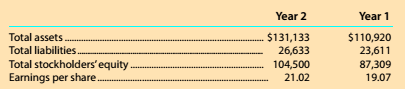Chapter 8, Problem 8.10.6MBA

Chapter
Section
Textbook Problem

Debt and price-earnings ratios Alphabet (formerly known as Google) (GOOG) is a technology company that offers users Internet search and e-mail services. Google also developed the Android operating system for use with cell phones and other mobile devices. The following data (in millions) were adapted from a recent financial statement of Alphabet.With a market price of \$526.40. compute the price-earnings ratio for Year 2.

To determine

Concept Introduction:

Price Earnings Ratio:

The price earnings ratio shows the relationship between price of the share and earnings per share. It is calculated with the help of following formula:

Price Earnings Ratio=Market price per shareEarnings per share

To Calculate:

The Price Earnings ratio for year 2

Explanation

The Price Earnings ratio for year 2 is calculated as follows:

Price Earnings Ratio=Market&#

Still sussing out bartleby?

Check out a sample textbook solution.

See a sample solution

The Solution to Your Study Problems

Bartleby provides explanations to thousands of textbook problems written by our experts, many with advanced degrees!

Get Started